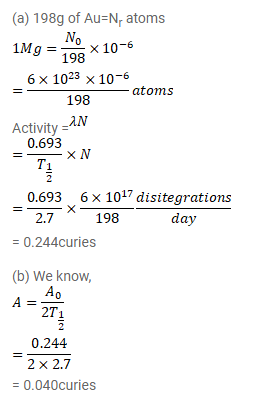# The half-life of 108Au is 2.7 days. (a) Find the activity of a sample

Question:

The half-life of ${ }^{108} \mathrm{Au}$ is $2.7$ days. (a) Find the activity of a sample containing $1.00 \mu \mathrm{g}$ of ${ }^{198} \mathrm{Au}$. (b) What will be the activity after 7 days? Take the atomic weight of ${ }^{198}$ Au to be $198 \mathrm{~g} / \mathrm{mol}$.

Solution: